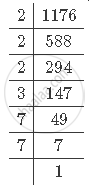Share

# If 1176 = 2^Axx3^Bxx7^C, Find the Values of A, B and C. Hence, Compute the Value of 2^Axx3^Bxx7^-c as a Fraction. - Mathematics

Course
ConceptLaws of Exponents for Real Numbers

#### Question

If 1176 = 2^axx3^bxx7^c, find the values of a, b and c. Hence, compute the value of 2^axx3^bxx7^-c as a fraction.

#### Solution

First find the prime factorisation of 1176.It can be observed that 1176 can be written as 2^3xx3^1xx7^2

1176=2^a3^b7^c=2^3 3^1 7^2

So, a = 3, b = 1 and c = 2.

Therefore, the value of 2^a xx3^bxx76-c is 2^3xx3^1xx7^-2=8xx3xx1/49=24/49

Is there an error in this question or solution?

#### Video TutorialsVIEW ALL 

Solution If 1176 = 2^Axx3^Bxx7^C, Find the Values of A, B and C. Hence, Compute the Value of 2^Axx3^Bxx7^-c as a Fraction. Concept: Laws of Exponents for Real Numbers.
S